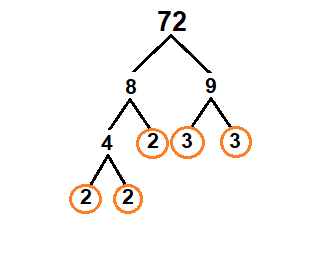# Factors of 72 | Prime Factorization of 72

The factors of 72 are the integers which divide 72 exactly, without leaving a remainder. Here are the factors of 72: 1, 2, 3, 4, 6, 8, 9, 12, 18, 24, 36, 72.

## What Are The Factors Of 72?

The factors of 72 are the integers that can be multiplied together to give the product 72. These factors are 1, 2, 3, 4, 6, 8, 9, 12, 18, 24, 36, and 72. Each of these numbers divides 72 without leaving a remainder. In terms of prime factorization, 72 can be written as 2^3 * 3^2, indicating that its prime factors are 2 and 3. Among the factors of 72, the numbers 2 and 3 are prime factors, while the rest are composite, except for 1, which is neither prime nor composite.

The factors can be paired as follows: (1, 72), (2, 36), (3, 24), (4, 18), (6, 12), and (8, 9). Each pair, when multiplied, equals 72.

## Prime Factorization of 72

Prime factorization of 72 is (2^3 × 3^2), meaning that 72 can be represented as the product of the prime numbers 2 and 3, raised to the powers of 3 and 2, respectively. Another way to express this is (2 × 2 × 2 × 3 × 3). This representation shows that 72 is composed of the prime numbers 2 and 3, and it can be written as a product of these primes.

One way to find the prime factorization of a number is to use a factor tree. Starting with the number 72, you divide it by the smallest prime number that divides it, which is 2, resulting in 36. You then continue the process with 36 and subsequently with the resulting numbers, until only prime numbers are left. This method helps visualize the step-by-step breakdown of the original number into its prime components.

Another method is to repeatedly divide the number by its smallest prime divisor and continue the process with the quotient, until the quotient is a prime number. For 72, the smallest prime divisor is 2, and continuing this process results in the prime factorization (2^3 × 3^2).

This mathematical process is fundamental for various applications such as cryptography, computer algorithms, and more advanced mathematical theories. It is one of the key concepts taught in elementary number theory and continues to be a significant area of research in mathematics.

Also Check: Factors of 106

## Factors Of 72 In Pairs

Different factors of a number are paired with each other, which will give the original number when the numbers of these pairs are multiplied by each other.

### Positive Pair Factors Of 72

Pair factors of positive 72 are as follows

1 × 72 = 72  (1, 72)

2 ×  36 = 72 (2, 36)

3 ×  24 = 72 (3, 24)

4 × 18 = 72  (4, 18)

6 × 12 = 72  (6, 12)

8 × 9 = 72  (8, 9)

### Negative Pair Factors Of 72

Pair factors of negative 72 are as follows;

-1 × -72 = 72 (-1, -72)

-2 × -36 = 72 (-2, -36)

-3 × -24 = 72 (-3, -24)

-4 × -18 = 72 (-4, -18)

-6 × -12 = 72 (-6, -12)

-8 × -9 = 72 (-8, -9)

## Factor Tree Of 72Pic Credit: Study.com

Here we have this factor tree of 72 in which circled numbers are Prime factors of this number. 2 and 3 are the prime number that we obtained through this tree.

## Prime Factorization of 72 using Exponential Notation

To find the prime factorization of 72 using exponential notation, you first break down 72 into prime numbers. Here are the steps:

72=2×36=2×2×18=2×2×18=2×2×2×9=2×2×2×9=2×2×2×3×3=2×2×2×3×3

So, the prime factorization of 72 using exponential notation is ${2}^{3}×{3}^{2}$.